12 Static Equilibrium and Elasticity

12.3 Stress, Strain, and Elastic Modulus

Learning Objectives

By the end of this section, you will be able to:

• Explain the concepts of stress and strain in describing elastic deformations of materials
• Describe the types of elastic deformation of objects and materials

A model of a rigid body is an idealized example of an object that does not deform under the actions of external forces. It is very useful when analyzing mechanical systems—and many physical objects are indeed rigid to a great extent. The extent to which an object can be perceived as rigid depends on the physical properties of the material from which it is made. For example, a ping-pong ball made of plastic is brittle, and a tennis ball made of rubber is elastic when acted upon by squashing forces. However, under other circumstances, both a ping-pong ball and a tennis ball may bounce well as rigid bodies. Similarly, someone who designs prosthetic limbs may be able to approximate the mechanics of human limbs by modeling them as rigid bodies; however, the actual combination of bones and tissues is an elastic medium.

For the remainder of this chapter, we move from consideration of forces that affect the motion of an object to those that affect an object’s shape. A change in shape due to the application of a force is known as a deformation. Even very small forces are known to cause some deformation. Deformation is experienced by objects or physical media under the action of external forces—for example, this may be squashing, squeezing, ripping, twisting, shearing, or pulling the objects apart. In the language of physics, two terms describe the forces on objects undergoing deformation: stress and strain.

Stress is a quantity that describes the magnitude of forces that cause deformation. Stress is generally defined as force per unit area. When forces pull on an object and cause its elongation, like the stretching of an elastic band, we call such stress a tensile stress. When forces cause a compression of an object, we call it a compressive stress. When an object is being squeezed from all sides, like a submarine in the depths of an ocean, we call this kind of stress a bulk stress (or volume stress). In other situations, the acting forces may be neither tensile nor compressive, and still produce a noticeable deformation. For example, suppose you hold a book tightly between the palms of your hands, then with one hand you press-and-pull on the front cover away from you, while with the other hand you press-and-pull on the back cover toward you. In such a case, when deforming forces act tangentially to the object’s surface, we call them ‘shear’ forces and the stress they cause is called shear stress.

The SI unit of stress is the pascal (Pa). When one newton of force presses on a unit surface area of one meter squared, the resulting stress is one pascal: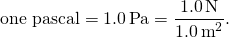In the British system of units, the unit of stress is ‘psi,’ which stands for ‘pound per square inch’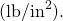Another unit that is often used for bulk stress is the atm (atmosphere). Conversion factors are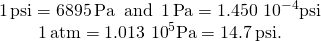An object or medium under stress becomes deformed. The quantity that describes this deformation is called strain. Strain is given as a fractional change in either length (under tensile stress) or volume (under bulk stress) or geometry (under shear stress). Therefore, strain is a dimensionless number. Strain under a tensile stress is called tensile strain, strain under bulk stress is called bulk strain (or volume strain), and that caused by shear stress is called shear strain.

The greater the stress, the greater the strain; however, the relation between strain and stress does not need to be linear. Only when stress is sufficiently low is the deformation it causes in direct proportion to the stress value. The proportionality constant in this relation is called the elastic modulus. In the linear limit of low stress values, the general relation between stress and strain is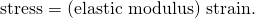As we can see from dimensional analysis of this relation, the elastic modulus has the same physical unit as stress because strain is dimensionless.

We can also see from (Figure) that when an object is characterized by a large value of elastic modulus, the effect of stress is small. On the other hand, a small elastic modulus means that stress produces large strain and noticeable deformation. For example, a stress on a rubber band produces larger strain (deformation) than the same stress on a steel band of the same dimensions because the elastic modulus for rubber is two orders of magnitude smaller than the elastic modulus for steel.

The elastic modulus for tensile stress is called Young’s modulus; that for the bulk stress is called the bulk modulus; and that for shear stress is called the shear modulus. Note that the relation between stress and strain is an observed relation, measured in the laboratory. Elastic moduli for various materials are measured under various physical conditions, such as varying temperature, and collected in engineering data tables for reference ((Figure)). These tables are valuable references for industry and for anyone involved in engineering or construction. In the next section, we discuss strain-stress relations beyond the linear limit represented by (Figure), in the full range of stress values up to a fracture point. In the remainder of this section, we study the linear limit expressed by (Figure).

Approximate Elastic Moduli for Selected Materials
Material Young’s modulus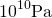Bulk modulusShear modulusAluminum 7.0 7.5 2.5
Bone (tension) 1.6 0.8 8.0
Bone (compression) 0.9
Brass 9.0 6.0 3.5
Brick 1.5
Concrete 2.0
Copper 11.0 14.0 4.4
Crown glass 6.0 5.0 2.5
Granite 4.5 4.5 2.0
Hair (human) 1.0
Hardwood 1.5 1.0
Iron 21.0 16.0 7.7
Marble 6.0 7.0 2.0
Nickel 21.0 17.0 7.8
Polystyrene 3.0
Silk 6.0
Steel 20.0 16.0 7.5
Acetone 0.07
Ethanol 0.09
Glycerin 0.45
Mercury 2.5
Water 0.22

Tensile or Compressive Stress, Strain, and Young’s Modulus

Tension or compression occurs when two antiparallel forces of equal magnitude act on an object along only one of its dimensions, in such a way that the object does not move. One way to envision such a situation is illustrated in (Figure). A rod segment is either stretched or squeezed by a pair of forces acting along its length and perpendicular to its cross-section. The net effect of such forces is that the rod changes its length from the original length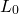that it had before the forces appeared, to a new length L that it has under the action of the forces. This change in length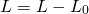may be either elongation (when L is larger than the original length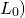or contraction (when L is smaller than the original length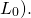Tensile stress and strain occur when the forces are stretching an object, causing its elongation, and the length change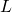is positive. Compressive stress and strain occur when the forces are contracting an object, causing its shortening, and the length changeis negative.

In either of these situations, we define stress as the ratio of the deforming force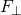to the cross-sectional area A of the object being deformed. The symbolthat we reserve for the deforming force means that this force acts perpendicularly to the cross-section of the object. Forces that act parallel to the cross-section do not change the length of an object. The definition of the tensile stress is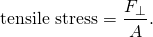Tensile strain is the measure of the deformation of an object under tensile stress and is defined as the fractional change of the object’s length when the object experiences tensile stress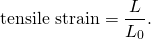Compressive stress and strain are defined by the same formulas, (Figure) and (Figure), respectively. The only difference from the tensile situation is that for compressive stress and strain, we take absolute values of the right-hand sides in (Figure) and (Figure).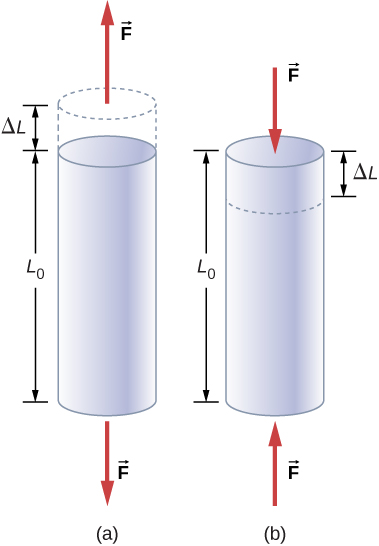Figure 12.18 When an object is in either tension or compression, the net force on it is zero, but the object deforms by changing its original length     (a) Tension: The rod is elongated by     (b) Compression: The rod is contracted by     In both cases, the deforming force acts along the length of the rod and perpendicular to its cross-section. In the linear range of low stress, the cross-sectional area of the rod does not change.

Young’s modulus Y is the elastic modulus when deformation is caused by either tensile or compressive stress, and is defined by (Figure). Dividing this equation by tensile strain, we obtain the expression for Young’s modulus: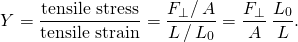Example

Compressive Stress in a Pillar

A sculpture weighing 10,000 N rests on a horizontal surface at the top of a 6.0-m-tall vertical pillar (Figure). The pillar’s cross-sectional area is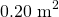and it is made of granite with a mass density of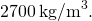Find the compressive stress at the cross-section located 3.0 m below the top of the pillar and the value of the compressive strain of the top 3.0-m segment of the pillar.Figure 12.19 Nelson’s Column in Trafalgar Square, London, England. (credit: modification of work by Cristian Bortes)

Strategy

First we find the weight of the 3.0-m-long top section of the pillar. The normal force that acts on the cross-section located 3.0 m down from the top is the sum of the pillar’s weight and the sculpture’s weight. Once we have the normal force, we use (Figure) to find the stress. To find the compressive strain, we find the value of Young’s modulus for granite in (Figure) and invert (Figure).

Solution

The volume of the pillar segment with height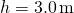and cross-sectional area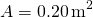is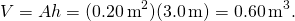With the density of granite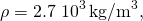the mass of the pillar segment is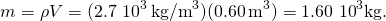The weight of the pillar segment is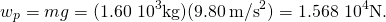The weight of the sculpture is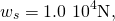so the normal force on the cross-sectional surface located 3.0 m below the sculpture is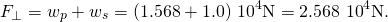Therefore, the stress is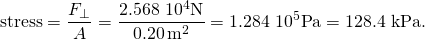Young’s modulus for granite is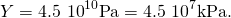Therefore, the compressive strain at this position is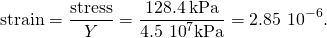Significance

Notice that the normal force acting on the cross-sectional area of the pillar is not constant along its length, but varies from its smallest value at the top to its largest value at the bottom of the pillar. Thus, if the pillar has a uniform cross-sectional area along its length, the stress is largest at its base.

Find the compressive stress and strain at the base of Nelson’s column.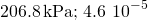Example

Stretching a Rod

A 2.0-m-long steel rod has a cross-sectional area of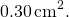The rod is a part of a vertical support that holds a heavy 550-kg platform that hangs attached to the rod’s lower end. Ignoring the weight of the rod, what is the tensile stress in the rod and the elongation of the rod under the stress?

Strategy

First we compute the tensile stress in the rod under the weight of the platform in accordance with (Figure). Then we invert (Figure) to find the rod’s elongation, using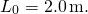From (Figure), Young’s modulus for steel is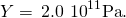Solution

Substituting numerical values into the equations gives us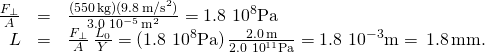Significance

Similarly as in the example with the column, the tensile stress in this example is not uniform along the length of the rod. Unlike in the previous example, however, if the weight of the rod is taken into consideration, the stress in the rod is largest at the top and smallest at the bottom of the rod where the equipment is attached.

A 2.0-m-long wire stretches 1.0 mm when subjected to a load. What is the tensile strain in the wire?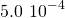Objects can often experience both compressive stress and tensile stress simultaneously (Figure). One example is a long shelf loaded with heavy books that sags between the end supports under the weight of the books. The top surface of the shelf is in compressive stress and the bottom surface of the shelf is in tensile stress. Similarly, long and heavy beams sag under their own weight. In modern building construction, such bending strains can be almost eliminated with the use of I-beams (Figure).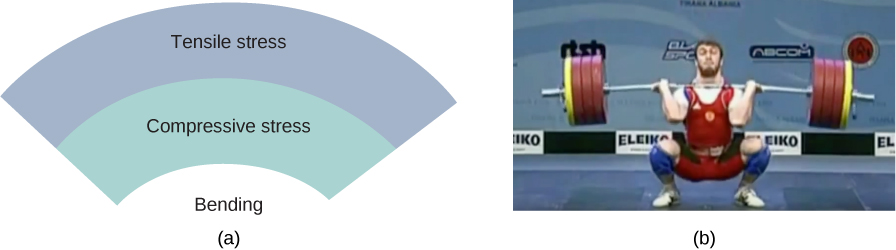Figure 12.20 (a) An object bending downward experiences tensile stress (stretching) in the upper section and compressive stress (compressing) in the lower section. (b) Elite weightlifters often bend iron bars temporarily during lifting, as in the 2012 Olympics competition. (credit b: modification of work by Oleksandr Kocherzhenko)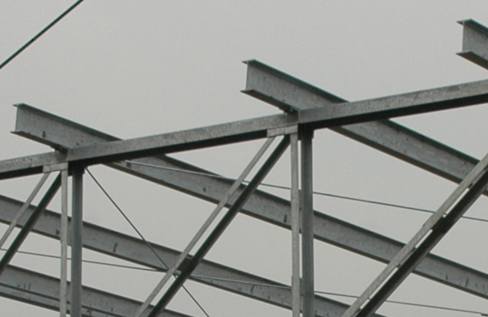Figure 12.21 Steel I-beams are used in construction to reduce bending strains. (credit: modification of work by “US Army Corps of Engineers Europe District”/Flickr)

A heavy box rests on a table supported by three columns. View this demonstration to move the box to see how the compression (or tension) in the columns is affected when the box changes its position.

Bulk Stress, Strain, and Modulus

When you dive into water, you feel a force pressing on every part of your body from all directions. What you are experiencing then is bulk stress, or in other words, pressure. Bulk stress always tends to decrease the volume enclosed by the surface of a submerged object. The forces of this “squeezing” are always perpendicular to the submerged surface (Figure). The effect of these forces is to decrease the volume of the submerged object by an amount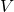compared with the volume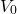of the object in the absence of bulk stress. This kind of deformation is called bulk strain and is described by a change in volume relative to the original volume: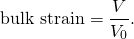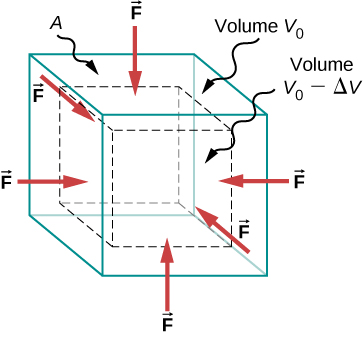Figure 12.22 An object under increasing bulk stress always undergoes a decrease in its volume. Equal forces perpendicular to the surface act from all directions. The effect of these forces is to decrease the volume by the amount     compared to the original volume,

The bulk strain results from the bulk stress, which is a forcenormal to a surface that presses on the unit surface area A of a submerged object. This kind of physical quantity, or pressure p, is defined as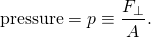We will study pressure in fluids in greater detail in Fluid Mechanics. An important characteristic of pressure is that it is a scalar quantity and does not have any particular direction; that is, pressure acts equally in all possible directions. When you submerge your hand in water, you sense the same amount of pressure acting on the top surface of your hand as on the bottom surface, or on the side surface, or on the surface of the skin between your fingers. What you are perceiving in this case is an increase in pressure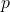over what you are used to feeling when your hand is not submerged in water. What you feel when your hand is not submerged in the water is the normal pressure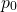of one atmosphere, which serves as a reference point. The bulk stress is this increase in pressure, or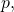over the normal level,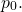When the bulk stress increases, the bulk strain increases in response, in accordance with (Figure). The proportionality constant in this relation is called the bulk modulus, B, or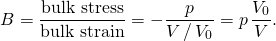The minus sign that appears in (Figure) is for consistency, to ensure that B is a positive quantity. Note that the minus sign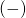is necessary because an increasein pressure (a positive quantity) always causes a decreasein volume, and decrease in volume is a negative quantity. The reciprocal of the bulk modulus is called compressibility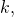or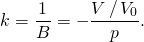The term ‘compressibility’ is used in relation to fluids (gases and liquids). Compressibility describes the change in the volume of a fluid per unit increase in pressure. Fluids characterized by a large compressibility are relatively easy to compress. For example, the compressibility of water is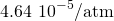and the compressibility of acetone is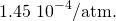This means that under a 1.0-atm increase in pressure, the relative decrease in volume is approximately three times as large for acetone as it is for water.

Example

Hydraulic Press

In a hydraulic press (Figure), a 250-liter volume of oil is subjected to a 2300-psi pressure increase. If the compressibility of oil is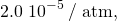find the bulk strain and the absolute decrease in the volume of oil when the press is operating.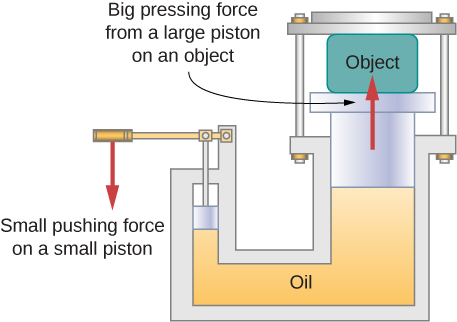Figure 12.23 In a hydraulic press, when a small piston is displaced downward, the pressure in the oil is transmitted throughout the oil to the large piston, causing the large piston to move upward. A small force applied to a small piston causes a large pressing force, which the large piston exerts on an object that is either lifted or squeezed. The device acts as a mechanical lever.

Strategy

We must invert (Figure) to find the bulk strain. First, we convert the pressure increase from psi to atm,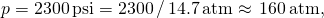and identify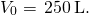Solution

Substituting values into the equation, we have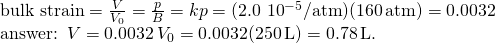Significance

Notice that since the compressibility of water is 2.32 times larger than that of oil, if the working substance in the hydraulic press of this problem were changed to water, the bulk strain as well as the volume change would be 2.32 times larger.

If the normal force acting on each face of a cubical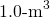piece of steel is changed by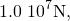find the resulting change in the volume of the piece of steel.

63 mL

Shear Stress, Strain, and Modulus

The concepts of shear stress and strain concern only solid objects or materials. Buildings and tectonic plates are examples of objects that may be subjected to shear stresses. In general, these concepts do not apply to fluids.

Shear deformation occurs when two antiparallel forces of equal magnitude are applied tangentially to opposite surfaces of a solid object, causing no deformation in the transverse direction to the line of force, as in the typical example of shear stress illustrated in (Figure). Shear deformation is characterized by a gradual shift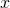of layers in the direction tangent to the acting forces. This gradation inoccurs in the transverse direction along some distance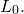Shear strain is defined by the ratio of the largest displacementto the transverse distance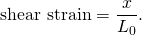Shear strain is caused by shear stress. Shear stress is due to forces that act parallel to the surface. We use the symbol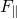for such forces. The magnitudeper surface area A where shearing force is applied is the measure of shear stress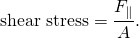The shear modulus is the proportionality constant in (Figure) and is defined by the ratio of stress to strain. Shear modulus is commonly denoted by S: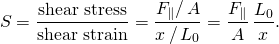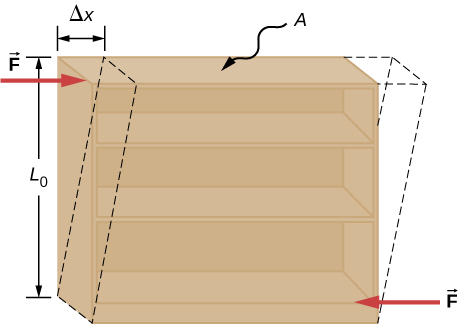Figure 12.24 An object under shear stress: Two antiparallel forces of equal magnitude are applied tangentially to opposite parallel surfaces of the object. The dashed-line contour depicts the resulting deformation. There is no change in the direction transverse to the acting forces and the transverse length     is unaffected. Shear deformation is characterized by a gradual shift     of layers in the direction tangent to the forces.

Example

An Old Bookshelf

A cleaning person tries to move a heavy, old bookcase on a carpeted floor by pushing tangentially on the surface of the very top shelf. However, the only noticeable effect of this effort is similar to that seen in (Figure), and it disappears when the person stops pushing. The bookcase is 180.0 cm tall and 90.0 cm wide with four 30.0-cm-deep shelves, all partially loaded with books. The total weight of the bookcase and books is 600.0 N. If the person gives the top shelf a 50.0-N push that displaces the top shelf horizontally by 15.0 cm relative to the motionless bottom shelf, find the shear modulus of the bookcase.

Strategy

The only pieces of relevant information are the physical dimensions of the bookcase, the value of the tangential force, and the displacement this force causes. We identify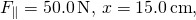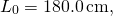and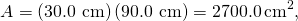and we use (Figure) to compute the shear modulus.

Solution

Substituting numbers into the equations, we obtain for the shear modulus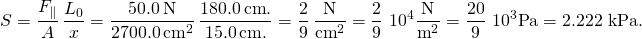We can also find shear stress and strain, respectively: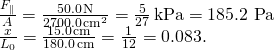Significance

If the person in this example gave the shelf a healthy push, it might happen that the induced shear would collapse it to a pile of rubbish. Much the same shear mechanism is responsible for failures of earth-filled dams and levees; and, in general, for landslides.

Explain why the concepts of Young’s modulus and shear modulus do not apply to fluids.

Fluids have different mechanical properties than those of solids; fluids flow.

Summary

• External forces on an object (or medium) cause its deformation, which is a change in its size and shape. The strength of the forces that cause deformation is expressed by stress, which in SI units is measured in the unit of pressure (pascal). The extent of deformation under stress is expressed by strain, which is dimensionless.
• For a small stress, the relation between stress and strain is linear. The elastic modulus is the proportionality constant in this linear relation.
• Tensile (or compressive) strain is the response of an object or medium to tensile (or compressive) stress. Here, the elastic modulus is called Young’s modulus. Tensile (or compressive) stress causes elongation (or shortening) of the object or medium and is due to an external forces acting along only one direction perpendicular to the cross-section.
• Bulk strain is the response of an object or medium to bulk stress. Here, the elastic modulus is called the bulk modulus. Bulk stress causes a change in the volume of the object or medium and is caused by forces acting on the body from all directions, perpendicular to its surface. Compressibility of an object or medium is the reciprocal of its bulk modulus.
• Shear strain is the deformation of an object or medium under shear stress. The shear modulus is the elastic modulus in this case. Shear stress is caused by forces acting along the object’s two parallel surfaces.

Conceptual Questions

Note: Unless stated otherwise, the weights of the wires, rods, and other elements are assumed to be negligible. Elastic moduli of selected materials are given in (Figure).

Why can a squirrel jump from a tree branch to the ground and run away undamaged, while a human could break a bone in such a fall?

In contact with the ground, stress in squirrel’s limbs is smaller than stress in human’s limbs.

When a glass bottle full of vinegar warms up, both the vinegar and the glass expand, but the vinegar expands significantly more with temperature than does the glass. The bottle will break if it is filled up to its very tight cap. Explain why and how a pocket of air above the vinegar prevents the bottle from breaking.

A thin wire strung between two nails in the wall is used to support a large picture. Is the wire likely to snap if it is strung tightly or if it is strung so that it sags considerably?

tightly

Review the relationship between stress and strain. Can you find any similarities between the two quantities?

What type of stress are you applying when you press on the ends of a wooden rod? When you pull on its ends?

compressive; tensile

Can compressive stress be applied to a rubber band?

Can Young’s modulus have a negative value? What about the bulk modulus?

no

If a hypothetical material has a negative bulk modulus, what happens when you squeeze a piece of it?

Discuss how you might measure the bulk modulus of a liquid.

Problems

The “lead” in pencils is a graphite composition with a Young’s modulus of approximately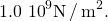Calculate the change in length of the lead in an automatic pencil if you tap it straight into the pencil with a force of 4.0 N. The lead is 0.50 mm in diameter and 60 mm long.

0.3 mm

TV broadcast antennas are the tallest artificial structures on Earth. In 1987, a 72.0-kg physicist placed himself and 400 kg of equipment at the top of a 610-m-high antenna to perform gravity experiments. By how much was the antenna compressed, if we consider it to be equivalent to a steel cylinder 0.150 m in radius?

By how much does a 65.0-kg mountain climber stretch her 0.800-cm diameter nylon rope when she hangs 35.0 m below a rock outcropping? (For nylon,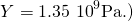9.0 cm

When water freezes, its volume increases by 9.05%. What force per unit area is water capable of exerting on a container when it freezes?

A farmer making grape juice fills a glass bottle to the brim and caps it tightly. The juice expands more than the glass when it warms up, in such a way that the volume increases by 0.2%. Calculate the force exerted by the juice per square centimeter if its bulk modulus is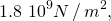assuming the bottle does not break.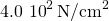A disk between vertebrae in the spine is subjected to a shearing force of 600.0 N. Find its shear deformation, using the shear modulus of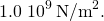The disk is equivalent to a solid cylinder 0.700 cm high and 4.00 cm in diameter.

A vertebra is subjected to a shearing force of 500.0 N. Find the shear deformation, taking the vertebra to be a cylinder 3.00 cm high and 4.00 cm in diameter. How does your result compare with the result obtained in the preceding problem? Are spinal problems more common in disks than in vertebrae?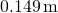Calculate the force a piano tuner applies to stretch a steel piano wire by 8.00 mm, if the wire is originally 1.35 m long and its diameter is 0.850 mm.

A 20.0-m-tall hollow aluminum flagpole is equivalent in strength to a solid cylinder 4.00 cm in diameter. A strong wind bends the pole as much as a horizontal 900.0-N force on the top would do. How far to the side does the top of the pole flex?

0.57 mm

A copper wire of diameter 1.0 cm stretches 1.0% when it is used to lift a load upward with an acceleration of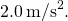What is the weight of the load?

As an oil well is drilled, each new section of drill pipe supports its own weight and the weight of the pipe and the drill bit beneath it. Calculate the stretch in a new 6.00-m-long steel pipe that supports a 100-kg drill bit and a 3.00-km length of pipe with a linear mass density of 20.0 kg/m. Treat the pipe as a solid cylinder with a 5.00-cm diameter.

8.59 mm

A large uniform cylindrical steel rod of density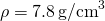is 2.0 m long and has a diameter of 5.0 cm. The rod is fastened to a concrete floor with its long axis vertical. What is the normal stress in the rod at the cross-section located at (a) 1.0 m from its lower end? (b) 1.5 m from the lower end?

A 90-kg mountain climber hangs from a nylon rope and stretches it by 25.0 cm. If the rope was originally 30.0 m long and its diameter is 1.0 cm, what is Young’s modulus for the nylon?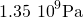A suspender rod of a suspension bridge is 25.0 m long. If the rod is made of steel, what must its diameter be so that it does not stretch more than 1.0 cm when a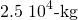truck passes by it? Assume that the rod supports all of the weight of the truck.

A copper wire is 1.0 m long and its diameter is 1.0 mm. If the wire hangs vertically, how much weight must be added to its free end in order to stretch it 3.0 mm?

259.0 N

A 100-N weight is attached to a free end of a metallic wire that hangs from the ceiling. When a second 100-N weight is added to the wire, it stretches 3.0 mm. The diameter and the length of the wire are 1.0 mm and 2.0 m, respectively. What is Young’s modulus of the metal used to manufacture the wire?

The bulk modulus of a material is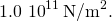What fractional change in volume does a piece of this material undergo when it is subjected to a bulk stress increase of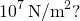Assume that the force is applied uniformly over the surface.

0.01%

Normal forces of magnitude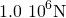are applied uniformly to a spherical surface enclosing a volume of a liquid. This causes the radius of the surface to decrease from 50.000 cm to 49.995 cm. What is the bulk modulus of the liquid?

During a walk on a rope, a tightrope walker creates a tension of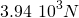in a wire that is stretched between two supporting poles that are 15.0 m apart. The wire has a diameter of 0.50 cm when it is not stretched. When the walker is on the wire in the middle between the poles the wire makes an angle of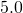below the horizontal. How much does this tension stretch the steel wire when the walker is this position?

1.44 cm

When using a pencil eraser, you exert a vertical force of 6.00 N at a distance of 2.00 cm from the hardwood-eraser joint. The pencil is 6.00 mm in diameter and is held at an angle of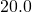to the horizontal. (a) By how much does the wood flex perpendicular to its length? (b) How much is it compressed lengthwise?

Normal forces are applied uniformly over the surface of a spherical volume of water whose radius is 20.0 cm. If the pressure on the surface is increased by 200 MPa, by how much does the radius of the sphere decrease?

0.63 cm

Glossary

bulk modulus
elastic modulus for the bulk stress
bulk strain
(or volume strain) strain under the bulk stress, given as fractional change in volume
bulk stress
(or volume stress) stress caused by compressive forces, in all directions
compressibility
reciprocal of the bulk modulus
compressive strain
strain that occurs when forces are contracting an object, causing its shortening
compressive stress
stress caused by compressive forces, only in one direction
elastic modulus
proportionality constant in linear relation between stress and strain, in SI pascals
normal pressure
pressure of one atmosphere, serves as a reference level for pressure
pascal (Pa)
SI unit of stress, SI unit of pressure
pressure
force pressing in normal direction on a surface per the surface area, the bulk stress in fluids
shear modulus
elastic modulus for shear stress
shear strain
strain caused by shear stress
shear stress
stress caused by shearing forces
strain
dimensionless quantity that gives the amount of deformation of an object or medium under stress
stress
quantity that contains information about the magnitude of force causing deformation, defined as force per unit area
tensile strain
strain under tensile stress, given as fractional change in length, which occurs when forces are stretching an object, causing its elongation
tensile stress
stress caused by tensile forces, only in one direction, which occurs when forces are stretching an object, causing its elongation
Young’s modulus
elastic modulus for tensile or compressive stress